# Mathematical statistics

2010 Mathematics Subject Classification: Primary: 62-01 [MSN][ZBL]

The branch of mathematics devoted to the study of mathematical methods for the organization, processing and utilization of statistical data for scientific and practical conclusions. Here, by statistical data is meant information on a number of objects in some, more or less extensive, collection, which have some specific properties.

## The object and method of mathematical statistics.

The statistical description of a collection of objects occupies an intermediate position between the individual description of each object in the collection, on the one hand, and the description of the collection by their common properties, with no individual breakdown into objects, on the other. By comparison with the first method, statistical data are always, to a greater or lesser extent, collective, and have only limited value in cases where the essence is the individual data (for example, a teacher getting to know a class obtains only a very preliminary orientation on the situation from the statistics on the number of excellent, good, adequate, and inadequate appraisals made by his or her predecessor). On the other hand, in comparison with data on a collection which is observed from the outside, and summarized by common properties, statistical data give a deeper penetration into the heart of the matter. For example, data on granulometric analysis of a rock (that is, data on the distribution of rock particles by size) gives valuable additional information when compared to measurements on the unfragmented form of the rock, which allows one, to some extent, to explain the properties of the rock, the conditions of its formation, etc.

The method of research, characterized as the discussion of statistical data on various collections of objects, is called statistical. The statistical method can be applied in very diverse areas of knowledge. However, the features of the statistical method in its applications to various kinds of objects are so specific that it would be meaningless to unify, for example, socio-economic statistics, physical statistics, stellar statistics, etc., in one science.

The common features of the statistical method in various areas of knowledge come down to the calculation of the number of objects in some group or other, the discussion of the distribution of quantitative attributes, the application of the sampling method (in cases where a detailed investigation of an extensive collection is difficult), the use of probability theory to estimate the adequacy of a number of observations for this or that conclusion, etc. This formal mathematical side of statistical research methods is indifferent to the specific nature of the objects being studied and comprises the topic of mathematical statistics.

## The connection between mathematical statistics and probability theory.

This connection is different in different cases. Probability theory studies not just any mass phenomenon, but phenomena which are random, to wit, "probabilistically random" . That is, those for which it makes sense to talk of associated probability distributions. Nevertheless, probability theory plays a definite role in the statistical study of mass phenomena of any kind, even those unrelated to the category of probabilistically random phenomena. This comes about through the theories of the sampling method and errors (cf. Errors, theory of; Sample method), which are based on probability theory. In these cases the phenomenon itself is not subject to probabilistic laws, but the means of investigation is.

A more important role is played by probability theory in the statistical investigation of probabilistically random phenomena. Here one finds in full measure the application of such probabilistically based parts of mathematical statistics as statistical hypotheses testing (cf. Statistical hypotheses, verification of), statistical estimation of probability distributions and their parameters, etc. The field of application of these deeper statistical methods is considerably narrower, since it is required that the phenomena themselves are subject to fairly definite probability laws. For example, the statistical study of turbulent regimes of water flow, or fluctuations in radio reception, is carried out on the basis of the theory of stationary stochastic processes. However, the application of this same theory to the analysis of economic time series may lead to gross errors, since the assumption of a time-invariant probability distribution in the definition of a stationary process is, as a rule, totally unacceptable in this case.

Probability laws gain a statistical expression on the strength of the law of large numbers (probabilities are realized approximately in the form of frequencies, and expectations in the form of averages).

## The simplest modes of statistical description.

A collection ofobjects being studied may, relative to some qualitative property, be divided into classes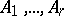. The statistical distribution corresponding to this partition is given by the numbers (frequencies)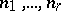(where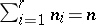) of objects in the different classes. Instead of the numberone often gives the corresponding relative frequency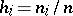(satisfying, obviously,). If the investigation concerns some quantitative attribute, then its distribution in the collection ofobjects may be given by directly listing the observed values of the attribute:; for example, in increasing order. However, for largesuch a method is cumbersome and, at the same time, does not clearly reveal the essential properties of the distribution. For arbitrarily large, in practice it is very unusual to compile complete tables of the observed values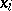, but rather to proceed in all subsequent work from tables which contain only the numbers in the classes obtained by grouping the observations into appropriate intervals.

Usually a grouping into 10–20 intervals, each containing no more thanto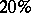of the values, turns out to be sufficient for a fairly complete classification of the essential properties of the distribution and for an appropriate computation, relative to the numbers in the groups, of the basic characteristics of the distribution (see below). Forming a histogram with respect to the grouped data graphically portrays the distribution. A histogram formed on the basis of groups with small intervals obviously has many peaks and does not graphically reflect the essential properties of the distribution. Number of parts. Diameter in mm.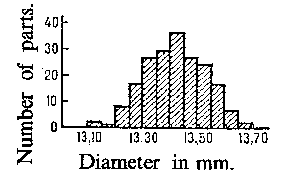Figure: m062710a

As an example, Fig. ais a histogram for the distribution of 200 diameters of certain parts (in mm), with group intervals of 0.05 mm, and Fig. bis the histogram of the same distribution with intervals of lengths 0.01 mm. Number of parts. Diameter in mm.Figure: m062710b

On the other hand, grouping into intervals which are too large may lead to a loss of clarity in the representation of the nature of the distribution, and to gross errors in the calculation of the mean and other characteristics of the distribution (see the corresponding histogram in Fig. c). Number of parts. Diameter in mm.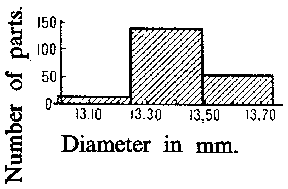Figure: m062710c

Within the limits of mathematical statistics, questions of grouping into intervals can only be considered from the formal point of view: the completeness of the mathematical description of a distribution, the precision of a calculation of means with respect to grouped data, etc.

The simplest summaries of the characteristics of the distribution of a single quantitative attribute are the mean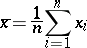and the mean-square deviation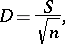where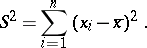In calculating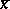,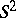andfor grouped data one uses the formulas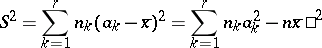orwhereis the number of grouped intervals and the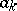are their means. If the material is grouped into intervals which are too large, then these calculations are too rough. Sometimes, in such cases it is useful to resort to special refinements of the classification. However, it only makes sense to introduce these refinements when definite probabilistic assumptions are satisfied.

Regarding the joint distribution of two or more attributes see Correlation (in statistics); Regression.

## The connection between statistical and probabilistic distributions. Parameter estimators. Testing probabilistic hypotheses.

Above, only certain selected simple modes of statistical description, which form a fairly extensive discipline with a well-developed system of ideas and techniques of calculation, were presented. Modes of statistical description, however, are of interest not just by themselves, but as a means of obtaining, from statistical material, inferences on the laws to which the phenomena studied are subject, and for obtaining inferences on the grounds leading in each individual case to various observed statistical distributions.

For example, the data drawn in Fig. a, Fig. band Fig. cwas collected with the aim of establishing the precision in the manufacturing of parts with design diameter equal to 13.40 mm under normal variations in manufacture. The simplest assumption, which may in this case be based upon some theoretical consideration, is that the diameters of the individual parts can be considered as a random variablesubject to the normal probability distribution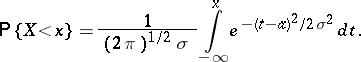(1)

If this assumption is true, then the parametersand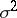— the mean and the variance of the probability distribution — can be fairly precisely estimated by the corresponding characteristics of the statistical distribution (since the number of observationsis sufficiently large). As an estimator of the theoretical variance it is preferred not to use the statistical variancebut the unbiased estimator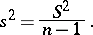For the theoretical mean-square deviationthere does not exist a single (suitable for any probability distribution) expression of an unbiased estimator. As an estimator (in general, biased) forit is most common to use. The accuracy of the estimatorsandforandis clarified by the corresponding variances, which, in the case of a normal distribution (1), have the form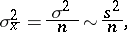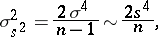where the signdenotes "approximate equality for large n" . Thus, if one agrees to add to the estimatorstheir mean-square deviation, one has for large, under the assumption of a normal distribution (1),(2)

The sample size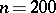is sufficient for the use in these formulas of laws from the theory of large samples.

For more information on the estimation of the parameters of theoretical probability distributions see Statistical estimation; Confidence estimation.

All rules based on probability theory for the statistical estimation of parameters and hypotheses testing operate only at a definite significance level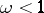, that is, they may lead to false results with probability. For example, if, under the assumption of a normal distribution and known theoretical variance, an interval estimator ofbased onis produced by the rulethen the probability of an error will be equal to, which is related tothrough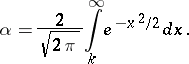The question of a rational choice of the significance level under given concrete conditions (for example, in the development of rules for statistical quality control in mass production) is very essential. In this connection the desire to apply only rules with a very high (close to 1) significance level faces the situation that for a restricted number of observations such rules only allow inferences with poor precision (it may not be possible to establish the inequality of probabilities even given a noticeable inequality of the frequencies, etc.).

## Further problems in mathematical statistics.

The above-mentioned methods of parameter estimation and hypotheses testing are based on the assumption that the number of observations required to attain a given precision in the conclusions is determined in advance (before carrying out the sampling). However, frequently an a priori determination of the number of observations is inconvenient, since by not fixing the number of trials in advance, but by determining it during the experiment, it is possible to decrease the expected number of trials. This situation was first observed in the example of choosing between one of two hypotheses in a sequence of independent trials. The corresponding procedure (first proposed in connection with problems of statistical sampling) is as follows: at each step decide, by the results of the observations already carried out, whether to a) conduct the next trial, or b) stop the trials and accept the first hypothesis, or c) stop the trials and accept the second hypothesis. With an appropriate choice of the quantitative characteristics such a procedure can secure (with the same precision in the calculations) a reduction in the average number of observations to almost half that of the fixed size sampling procedure (see Sequential analysis). The development of the methods of sequential analysis led, on the one hand, to the study of controlled stochastic processes (cf. Controlled stochastic process) and, on the other, to the appearance of statistical decision theory. This theory arises because the results of sequentially carrying out observations serve as a basis for the adoption of certain decisions (intermediate — to continue the trial, and final — when the trials are stopped). In problems on parameter estimation the final decisions are numbers (the values of the estimators), in problems on hypotheses testing they are the accepted hypothesis. The aim of the theory is to give rules for the acceptance of decisions which minimise the mean loss or risk (the risk depends on the probability distributions of the results of the observations, on the final decision, on the expense of conducting the trials, etc.).

Questions on the expedient distribution of effort in carrying out a statistical analysis of phenomena are considered in the theory of design of experiments, which plays a major part in modern mathematical statistics.

Side by side with the development and elaboration of the general ideas of mathematical statistics there have evolved various specialized branches such as dispersion analysis; covariance analysis; multi-dimensional statistical analysis; the statistical analysis of stochastic processes; and factor analysis. New considerations in regression analysis have appeared (see also Stochastic approximation). A major part in problems of mathematical statistics is played by the Bayesian approach to statistical problems.

## Historical information.

The first elements of mathematical statistics can already be found in the writings of the originators of probability theory — J. Bernoulli, P. Laplace and S. Poisson. In Russia the methods of mathematical statistics in the application to demography and actuarial work were developed by V.Ya. Bunyakovskii (1846). Of key importance for all subsequent development of mathematical statistics was the work of the classical Russian school of probability theory in the second half of the 19th century and beginning of the 20th century (P.L. Chebyshev, A.A. Markov, A.M. Lyapunov, and S.N. Bernshtein). Many questions of statistical estimation theory were essentially devised on the basis of the theory of errors and the method of least squares (C.F. Gauss and Markov). The work of A. Quételet, F. Galton and K. Pearson has great significance, but in terms of utilizing the achievements of probability theory they lagged behind that of the Russian school. Pearson widely expanded the work on the formation of tables of functions necessary for applying the methods of mathematical statistics. This important work was continued in many scientific centres (in the USSR it was carried out by E.E. Slutskii, N.V. Smirnov and L.N. Bol'shev). In the creation of small sample theory, the general theory of statistical estimation and hypotheses testing (free of assumptions on the presence of a priori distributions), and sequential analysis, the role of the Anglo-American school (Student, the pseudonym of W.S. Gosset, R.A. Fisher, Pearson, and J. Neyman), whose activity began in the 1920's, was very significant. In the USSR noteworthy results in the field of mathematical statistics were obtained by V.I. Romanovskii, A.N. Kolmogorov and Slutskii, to whom belongs important work on the statistics of dependent stationary series, Smirnov, who laid the foundations of the theory of non-parametric methods in statistics, and Yu.V. Linnik, who enriched the analytical apparatus of mathematical statistics with new methods. On the basis of mathematical statistics, statistical methods of research and investigation in queueing theory, physics, hydrology, climatology, stellar astronomy, biology, medicine, etc., were particularly intensively developed.

See also the references to the articles on branches of mathematical statistics.

How to Cite This Entry:
Mathematical statistics. Encyclopedia of Mathematics. URL: http://encyclopediaofmath.org/index.php?title=Mathematical_statistics&oldid=21661
This article was adapted from an original article by A.N. KolmogorovYu.V. Prokhorov (originator), which appeared in Encyclopedia of Mathematics - ISBN 1402006098. See original article